# Two Lines Of Symmetry

In Maths, the line of symmetry is the line that passes through the center of the shape/object and divides the shape into identical halves. It is an imaginary line or axis of the object. The line of symmetry is also known as the axis of symmetry or the mirror line. We are already acquainted with the term symmetry, which is a balanced and proportionate similarity found in two halves of an object; that is, one half is the mirror image of the other half. In this article, we are going to learn what is a line of symmetry, two lines of symmetry and the shapes having two lines of symmetry with examples.

## What is Line of Symmetry?

Let us take up an activity to understand the line of symmetry. Say you are asked to carve a ‘heart’ out of a piece of paper. For the heart to be perfectly symmetrical, you fold the paper into half and draw half of the heart along the fold. After cutting and opening the fold, you find the symmetrical heart. The vertical fold along which you made the heart is the line of symmetry.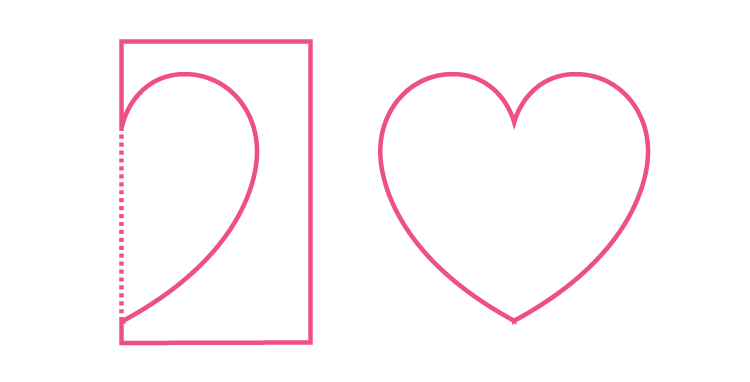Now try to find some other fold along which the perfectly symmetrical heart could have been cut out. You wouldn’t find any, would you? It can be concluded here that the heart shape has only 1 line of symmetry. We have already seen the example of a shape with one line of symmetry. However, figures with more than one line of symmetry also exist. In fact, a shape may have:

• No line of symmetry, which implies that the figure is asymmetrical
• One line of symmetry
• Two lines of symmetry
• Multiple (more than two) lines of symmetry.

For now, let us focus on shapes with two lines of symmetry.

## Two Lines of Symmetry

If the shapes can be divided into two equal parts with two lines, then the shapes are said to have two lines of symmetry. The line of symmetry can be a horizontal line of symmetry, vertical line of symmetry or diagonal line of symmetry.

### Two Lines of Symmetry Shapes

Now, let us have a look at some of the shapes having two lines of symmetry.

• A rectangle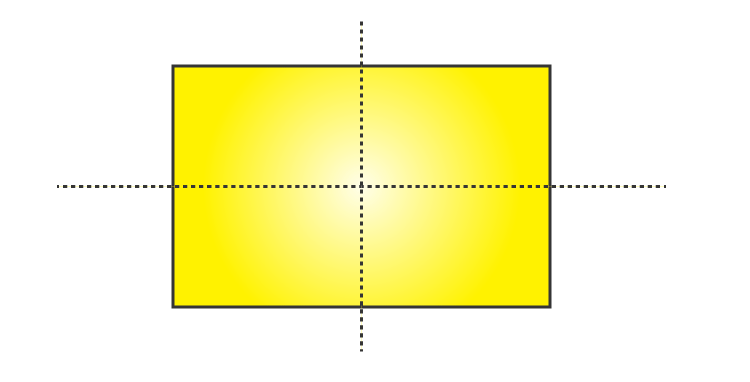• A rhombus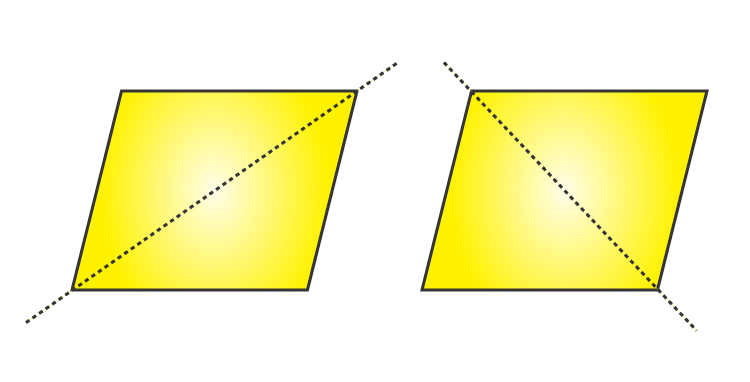• Alphabets like H and X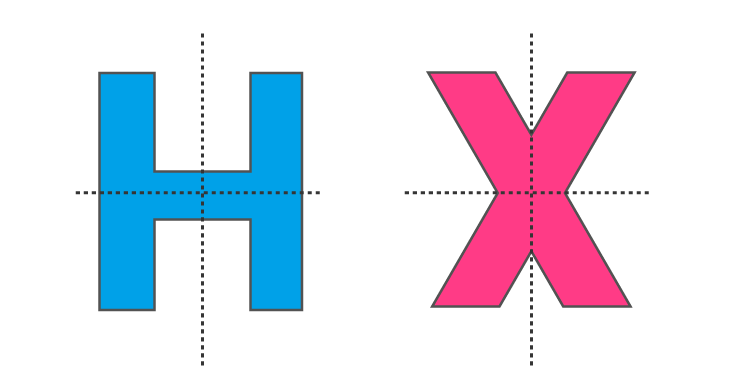• An hourglassA Cut Out From Double Fold

Let us do an activity to obtain a figure with two lines of symmetry.

Take an A4-sized sheet of paper. Fold it into two halves, say along the vertical. Fold it again, vertical this time. Draw some design along the folds and cut it out. Open the fold. The shape you obtain by following this procedure will have at least 2 lines of symmetry.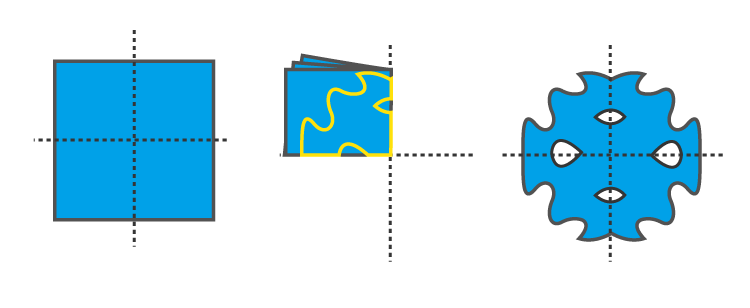### Examples

Determine the number of lines of symmetry for the following figures:

1.2.3.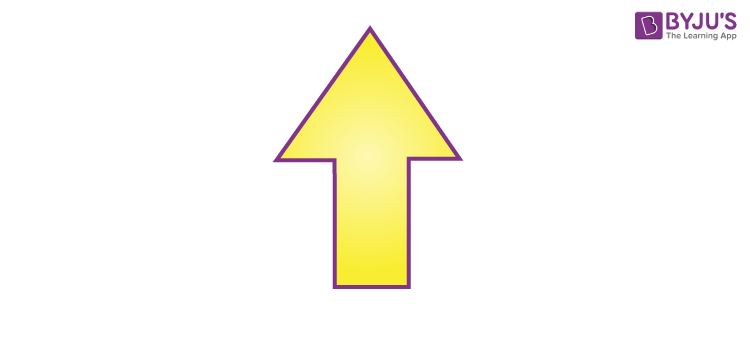## Frequently Asked Questions on Two Lines of Symmetry

Q1

### What is meant by two lines of symmetry?

A figure is said to have two lines of symmetry if a figure can be divided into two equal parts with two lines.

Q2

### Mention three capital letters of the English alphabets which have two lines of symmetry?

The three capital letters of English alphabets having two lines of symmetry are H, I, X.

Q3

### Does an equilateral triangle have two lines of symmetry?

No, an equilateral triangle does not have 2 lines of symmetry. Since all the three sides are equal in an equilateral triangle, it has three lines of symmetry.

Q4

### How many lines of symmetry does a rhombus have?

A rhombus has two lines of symmetry.

Q5

### Mention two different lines of symmetry?

The two different lines of symmetry are:
Horizontal line of symmetry
Vertical line of symmetry